# Differential Equation for a Pendulum

SilverSoldier
Homework Statement:
Suppose we have a simple pendulum, let's say with mass ##m##, attached to a massless string of length ##l##, on an ##xy## coordinate plane as indicated in the diagram below. I want to model the motion of this pendulum over time.
Relevant Equations:
##\tau=I\alpha##
Centripetal force = ##\dfrac{mv^2}{r}##
Potential energy = ##mgh##
Kinetic energy = ##\dfrac{1}{2}mv^2##
Conservation of energy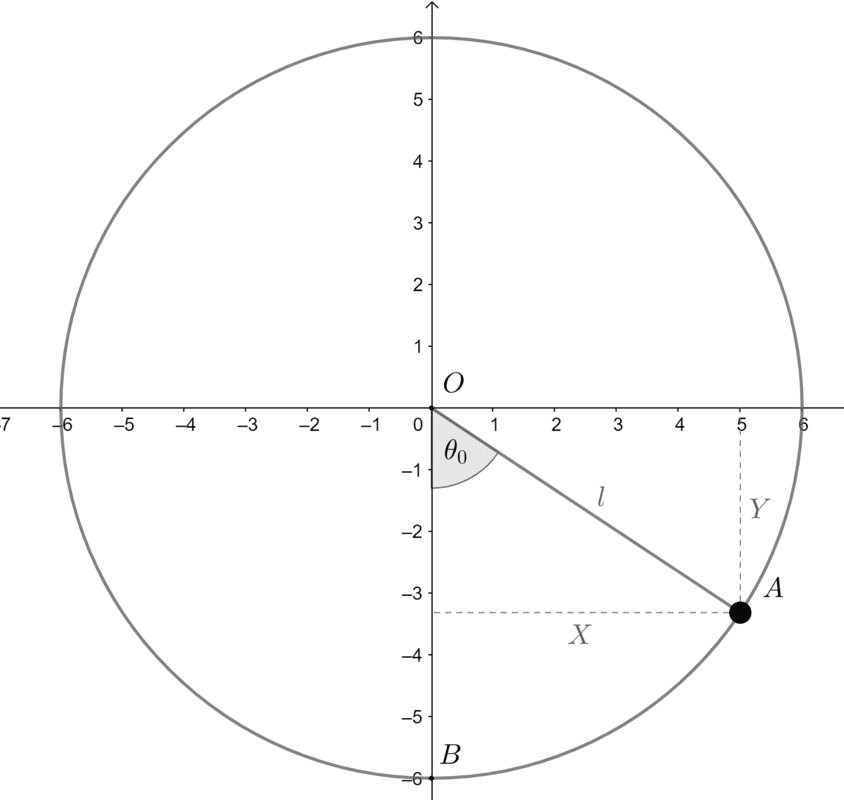Suppose we displace the pendulum bob ##A## an angle ##\theta_0## initially, and let go.
This is equivalent to giving it an initial horizontal displacement of ##X## and an initial vertical displacement of ##Y##. Let ##Y## initially be a negative number, and ##X## initially be positive.
I observe that in this initial state, it has some potential energy ##mg(l+Y)## with respect to point ##B##. This energy must be conserved.

Next, we look at some such general position as ##A'## of the pendulum bob, where its horizontal and vertical displacements are ##x## and ##y## respectively (##y<0##), as shown below. Let us suppose it has a net velocity ##v## at this instant. Let ##\theta## be the angle it forms with the vertical, and consider this angle to be negative.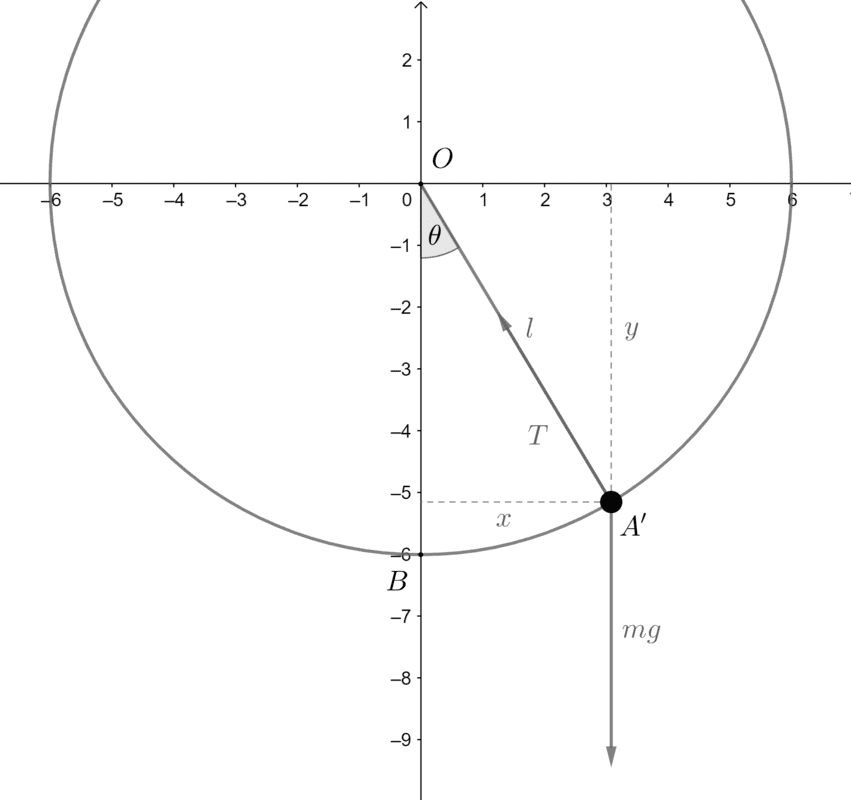Here, the bob would have lesser potential energy, and the difference, will have converted into its kinetic energy. So we have
\begin{align*} mg(l+Y)&=mg(l+y)+\dfrac{1}{2}mv^2\\ v^2&=2g(Y-y). \end{align*}
Now this pendulum is also undergoing non-uniform circular motion, so there must be a centripetal force directed towards the center to keep it going in the circle. This force must be provided by the tension force ##T## and the component of the weight force along the string. Therefore,
\begin{align*} T-mg\cos\theta&=\dfrac{mv^2}{l}\\ T&=mg\cos\theta+\dfrac{mv^2}{l}. \end{align*}
We know ##v^2## from the Principle of Conservation of Energy, so
$$T=mg\cos\theta+\dfrac{2mg(Y-y)}{l}.$$
Now, we resolve the forces on the pendulum bob vertically and horizontally, and use Newton's Second Law to find the accelerations in these directions. If the net horizontal acceleration in the right direction is ##a_x##, and net vertical force in the upward direction is ##a_y##, we have
\begin{align*} a_x&=\dfrac{T\sin\theta}{m}\\ a_x&=g\cos\theta\sin\theta+\dfrac{2g\sin\theta (Y-y)}{l}, \end{align*}
and
\begin{align*} a_y&=\dfrac{T\cos\theta-mg}{m}\\ a_y&=g\cos^2\theta+\dfrac{2g\cos\theta (Y-y)}{l}-g\\ &=\dfrac{2g\cos\theta (Y-y)}{l}-g\sin^2\theta. \end{align*}
We can also say that ##\sin\theta=\dfrac{x}{l}## and ##\cos\theta=-\dfrac{y}{l}##.

The net horizontal and vertical accelerations ##a_x## and ##a_y## that the object experiences, in terms of its position ##(x,y)## are therefore
$$a_x=\dfrac{gx}{l^2}\left(2Y-3y\right)$$
and
$$a_y=-\dfrac{g}{l^2}\left(x^2+2y(Y-y)\right).$$
Is this correct?

I'm not too sure what your endgame is here. Perhaps it is easier to consider the motion in terms of ##\theta##. By energy considerations, $$-mlg \cos{\theta_0} = -mlg \cos{\theta} + \frac{1}{2}ml^2 \dot{\theta}^2$$ $$\frac{2g}{l}[\cos{\theta} - \cos{\theta_0}] = \left(\frac{d\theta}{dt}\right)^2$$ There are a few things you might want to do with this. For instance, $$\sqrt{\frac{l}{2g}} \int_{0}^{\theta_0} \frac{d\theta}{\sqrt{\cos{\theta} - \cos{\theta_0}}} = \int_0^{\frac{T}{4}} dt = \frac{T}{4}$$ $$T = \sqrt{\frac{l}{g}} \times 2\sqrt{2} \int_{0}^{\theta_0} \frac{d\theta}{\sqrt{\cos{\theta} - \cos{\theta_0}}}$$ Or in other words, we've some sort of oscillatory motion with a time period which you can get if you compute whatever that thing turns out to be for some ##\theta_{0}##.

I'm not sure if you can do anything analytically (if you can then I don't know anything about it!). I don't believe you can get ##\theta(t)## without the small angle approximation, though I could be mistaken.

#### Attachments

Last edited by a moderator:
•SilverSoldier
Homework Helper
Gold Member
2022 Award
Is this correct?

Interesting! Probably not many people have thought of doing it that way before. Generally, you would do a problem like this in polar coordinates, and then transform to Cartesian if you want to. Note that the pendulum has only one "degree of freedom". That is, to move in the ##\theta## direction. That's why there was the suggestion above to look at motion in terms of a single variable ##\theta##.

You've done some hard work there, but it would be easier in polar coordinates.

•SilverSoldier and etotheipi
SilverSoldier
Generally, you would do a problem like this in polar coordinates, and then transform to Cartesian if you want to.

Wouldn't these be the same results obtained that way too?

I am kind of thinking of deriving an acceleration vector field, where I can obtain the acceleration that the bob undergoes in terms of the position it is at.

Wouldn't these be the same results obtained that way too?

I am kind of thinking of deriving an acceleration vector field, where I can obtain the acceleration that the bob undergoes in terms of the position it is at.

I just wonder how easy the transformation is. You've got two equations for the ##x## and ##y## accelerations in terms of position but it seems like it'll be pretty hard to transform that into position against time.

But best wait for the oracle (who also goes by the name @PeroK) to reply, since I've got no idea from here on!

•SilverSoldier
Homework Helper
Gold Member
2022 Award
Wouldn't these be the same results obtained that way too?

I am kind of thinking of deriving an acceleration vector field, where I can obtain the acceleration that the bob undergoes in terms of the position it is at.
For circular motion the acceleration in polar coordinates is:
$$a_r = -l \dot \theta^2, \ a_{\theta} = l \ddot \theta$$
We can get ##\dot \theta^2## from potential energy:
$$l^2 \dot \theta^2 = 2g(Y-y)$$
Hence:
$$a_r = - \frac{2g(Y-y)}{l}$$
And:
$$a_{\theta} = -g \sin \theta$$
Then you can transform these to Cartesian coordinates if you wish.

Last edited:
•etotheipi
SilverSoldier
$$l\dot{\theta}^2=2g(Y−y)$$

You probably meant ##l\dot{\theta}^2=\dfrac{2g(Y-y)}{l}##?

But converting these vectors into Cartesian coordinates give the same results I have obtained in the above equations.

What I would like to know is, can I define a vector field ##F## that looks like
$$F(x,y)=\dfrac{gx}{l^2}(2Y-3y)\hat{i}-\left(\dfrac{g}{l^2}(x^2+2y(Y-y))\right)\hat{j}\text{ ?}$$

Homework Helper
Gold Member
2022 Award
But converting these vectors into Cartesian coordinates give the same results I have obtained in the above equations.

But the solution in polar coordinates is much simpler. It respects the symmetry of the problem.

What I would like to know is, can I define a vector field ##F## that looks like
$$F(x,y)=\dfrac{gx}{l^2}(2Y-3y)\hat{i}-\left(\dfrac{g}{l^2}(x^2+2y(Y-y))\right)\hat{j}\text{ ?}$$

With what purpose?

SilverSoldier
With what purpose?

I am trying to model the motion of a pendulum in space. My approach is as follows.

I choose an initial position for the pendulum given by the Cartesian coordinates ##(X,Y)##, and have a computer program evaluate its horizontal and vertical accelerations at that position.

I then choose some small time interval ##t##, (say about ##0.001\text{ s}##), and multiply each the horizontal and vertical accelerations by ##t##, to get the change that must happen in the pendulum's horizontal and vertical velocities respectively at that instant. These velocity changes get added to variables storing its velocities.

I multiply the net velocities of the pendulum again by ##t## to get the changes that must happen in its displacement, horizontally and vertically.

These displacements are added to ##X## and ##Y## to get its new position, and the whole process repeats.

Clearly there must be errors in this process, but I believe it works well enough for small values of ##t##.

I am trying to model the motion of a pendulum in space. My approach is as follows.

I choose an initial position for the pendulum given by the Cartesian coordinates ##(X,Y)##, and have a computer program evaluate its horizontal and vertical accelerations at that position.

This stepwise method should work with either approach, cartesian or polar. Are you doing it on excel or something fancier? So long as the method you're using to do the simulation is numerical it should work out okay.

Homework Helper
Gold Member
2022 Award
I am trying to model the motion of a pendulum in space. My approach is as follows.

I choose an initial position for the pendulum given by the Cartesian coordinates ##(X,Y)##, and have a computer program evaluate its horizontal and vertical accelerations at that position.

I then choose some small time interval ##t##, (say about ##0.001\text{ s}##), and multiply each the horizontal and vertical accelerations by ##t##, to get the change that must happen in the pendulum's horizontal and vertical velocities respectively at that instant. These velocity changes get added to variables storing its velocities.

I multiply the net velocities of the pendulum again by ##t## to get the changes that must happen in its displacement, horizontally and vertically.

These displacements are added to ##X## and ##Y## to get its new position, and the whole process repeats.

Clearly there must be errors in this process, but I believe it works well enough for small values of ##t##.

That would be so much simpler in polar coordinates. You have the constraint of motion in a circle, so you need only model the one variable ##\theta## by the same differential method.

You have a relatively simple differential equation in ##\theta## to work with.

•etotheipi
SilverSoldier
We can also say that ##\sin\theta=\dfrac{x}{l}## and ##\cos\theta=-\dfrac{y}{l}##.

By the way, I just now noticed that we must have ##\sin\theta=-\dfrac{x}{l}##, because only then will the angle be treated negative in a region with positive ##x##🤦‍♂️, and all equations above must be negated appropriately. I have updated the program with these changes, by the way.

Are you doing it on excel or something fancier?

I am using a JavaScript program to animate the pendulum in an HTML <canvas> element.

It has worked quite well for other fields, and I am quite sure there is no error in the program, and it does seem to work well for small angles. For example, this is the path that is traced out by the pendulum when ##X=60## and ##Y=-60##. (All units are in pixels).But for ##X=60## and ##Y=-30##, this is the path I find is traced out by the pendulum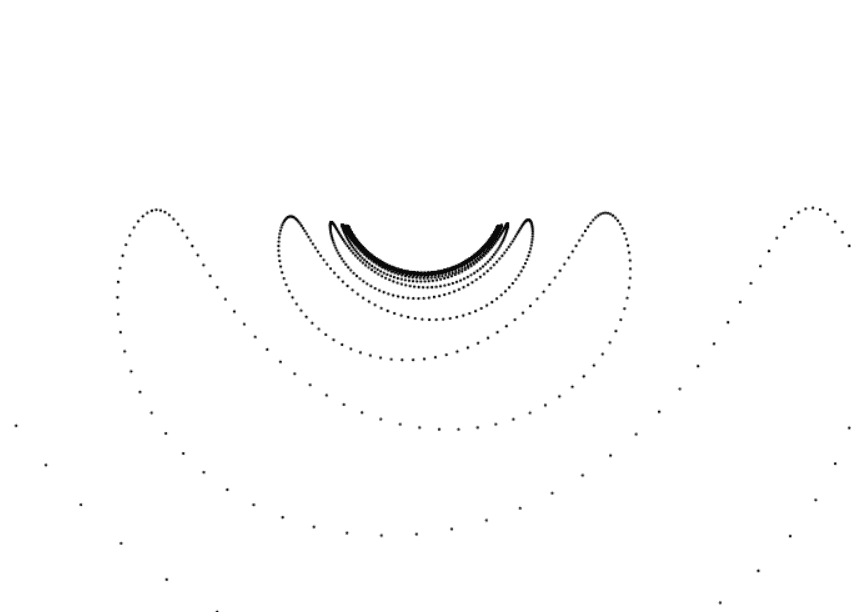I still believe this would happen with the previous case as well, if I look at it for long enough. Why might this be?

That would be so much simpler in polar coordinates. You have the constraint of motion in a circle, so you need only model the one variable ##\theta## by the same differential method.

You have a relatively simple differential equation in ##\theta## to work with.

Yes, I realize that, but I believe most phenomena I would like to model are best represented in Cartesian coordinates, rather than in polar coordinates#### Attachments

I wonder if you'd be happy to post your code? It might be easier to fix that way. Could be something as annoying as too small a step size, with small errors being exacerbated; have you tried lowering that?
Yes, I realize that, but I believe most phenomena I would like to model are best represented in Cartesian coordinates, rather than in polar coordinatesBut here it might be easiest to do the numerical solution with polar variables and then convert your points to cartesian form before you plot them. But if you've already done it in cartesian form then don't bother redoing it!

Homework Helper
Gold Member
2022 Award
Yes, I realize that, but I believe most phenomena I would like to model are best represented in Cartesian coordinates, rather than in polar coordinatesSilverSoldier
I wonder if you'd be happy to post your code? It might be easier to fix that way.
I believe this will do. I haven't included the part where the particle is actually drawn.
JavaScript:
var xVelocity = 0, yVelocity = 0, g = 1000;
var initX = 60;
var initY = -30;
var prevX = initX, prevY = initY;
var l = Math.sqrt(Math.pow(initX, 2) + Math.pow(initY, 2));

var time = 0.001; // The particle is made to move for this long

function f(x, y) {
var i = -((g*x)/Math.pow(l, 2))*(2*initY-3*y); // Coefficient of unit vector i
var j = -(g/Math.pow(l, 2))*(Math.pow(x, 2) + 2*initY*y - 2*Math.pow(y, 2)); // Coefficient of unit vector j
return {
i: i,
j: j,
slope: j/i,
magnitude: Math.sqrt(Math.pow(i, 2) + Math.pow(j, 2)),
};
}

function animateParticle() {
var output = f(prevX, prevY);

var xVelocityChange = output.i * time;
var yVelocityChange = output.j * time;

xVelocity += xVelocityChange;
yVelocity += yVelocityChange;

var xOffset = xVelocity * time;
var yOffset = yVelocity * time;

var newX = prevX + xOffset;
var newY = prevY + yOffset;

// Update prevX and prevY, so that the function is evaluated at the new values in the next iteration
prevX = newX;
prevY = newY;

window.requestAnimationFrame(animateParticle);
}

JavaScript:
var xOffset = xVelocity * time;
var yOffset = yVelocity * time;

This might well be a way to do it, but I wonder whether the incremental displacement should instead be quadratic in ##t##? As in
JavaScript:
var xOffset = xVelocity*time + 0.5*output.i*Math.pow(time, 2);

I wonder also whether this should go before you increment the velocities, I think it should.You've seen polar bears... now wait until you see the polar butterfly!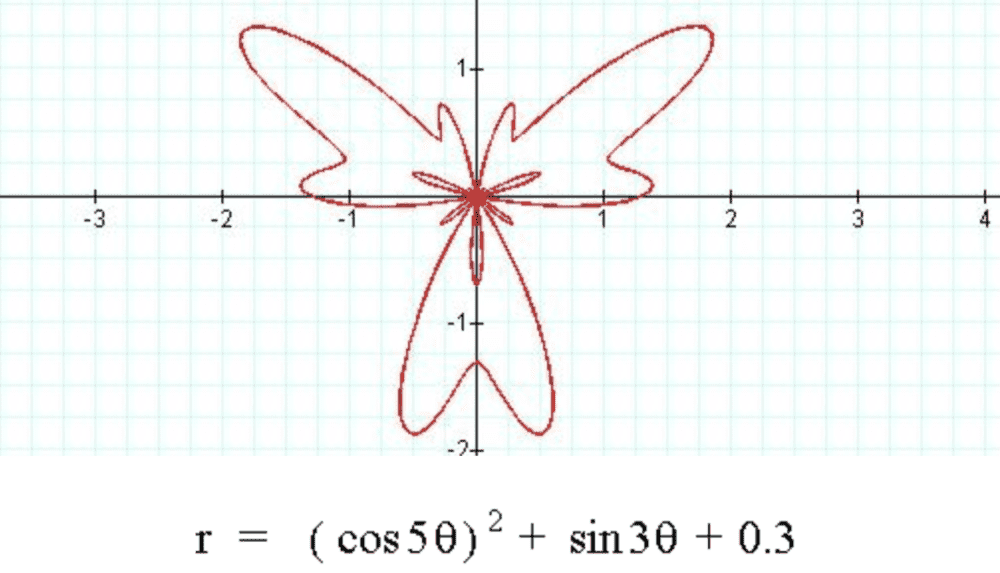•PeroK
Homework Helper
Gold Member
2022 Award
You've seen polar bears... now wait until you see the polar butterfly!

View attachment 261186
If there are polar bears, there must be Cartesian bears, I guess?

•etotheipi
If there are polar bears, there must be Cartesian bears, I guess?

Hmmmm...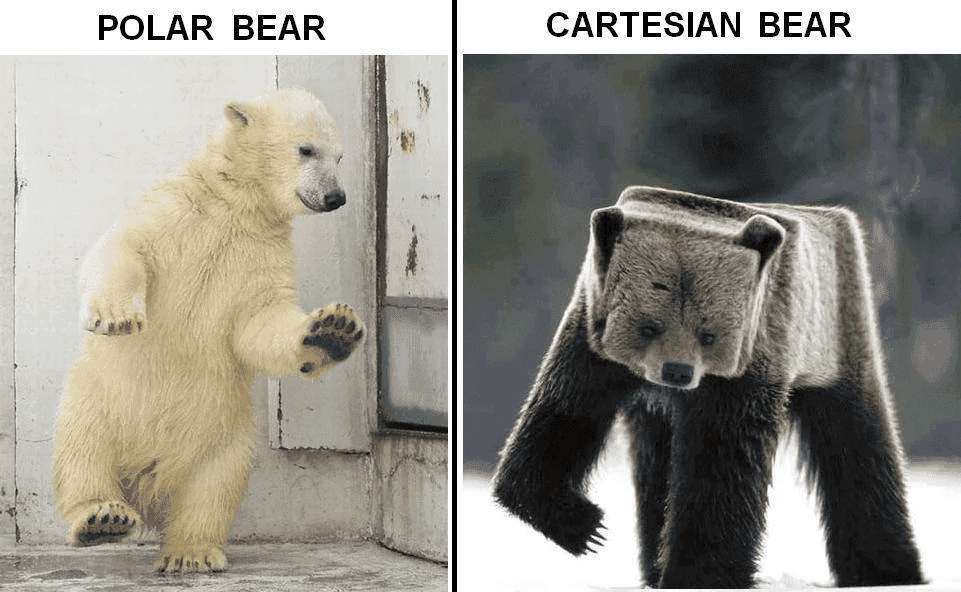•PeroK
SilverSoldier
JavaScript:
var xOffset = xVelocity * time;
var yOffset = yVelocity * time;

This might well be a way to do it, but I wonder whether the incremental displacement should instead be quadratic in ##t##? As in
JavaScript:
var xOffset = xVelocity*time + 0.5*output.i*Math.pow(time, 2);

I wonder also whether this should go before you increment the velocities, I think it should.

Thank you very much for these suggestions. But I get the following with
JavaScript:
var xOffset = xVelocity*time + (output.i*Math.pow(time, 2))/2;
var yOffset = yVelocity*time + (output.j*Math.pow(time, 2))/2;

xVelocity += xVelocityChange;
yVelocity += yVelocityChange;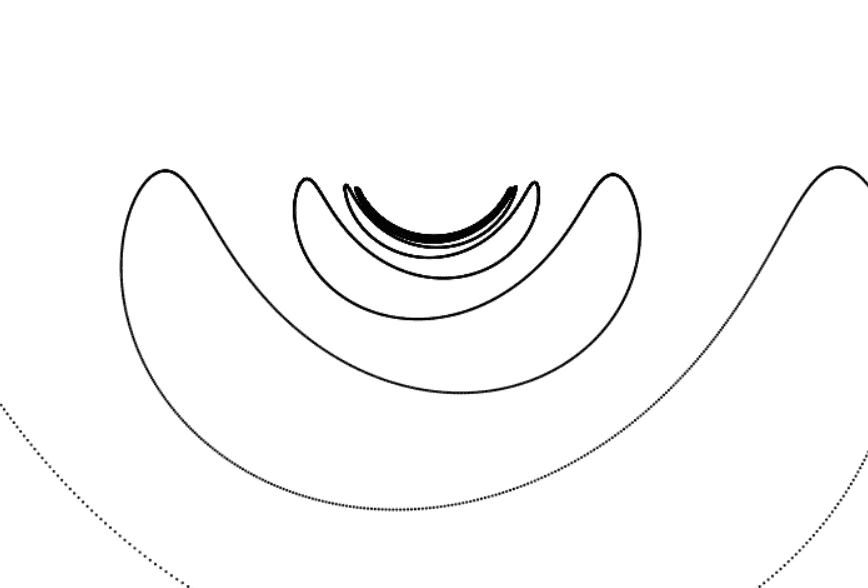With
JavaScript:
xVelocity += xVelocityChange;
yVelocity += yVelocityChange;

var xOffset = xVelocity*time + (output.i*Math.pow(time, 2))/2;
var yOffset = yVelocity*time + (output.j*Math.pow(time, 2))/2;
I get this.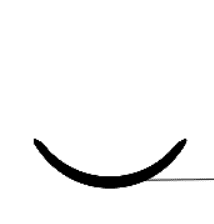This appears to be an improvement 🤷‍♂️, but it's not a single line---notice how the line is quite thick. It initially moved towards the center, and then moved outwards again. Can this happen?

That's kinda peculiar behaviour, if in your ##\delta t = 0.001## you can approximate the acceleration to be constant, then you should have ##v_x = u_x + a_x\delta t## and ##\delta x = u_x \delta t + \frac{1}{2}a_x {\delta t}^2##. In each case the ##u_x## is xVelocity before increment by ##a_x \delta t##. But if you've got it to work like that, then who am I to complain!
This appears to be an improvement 🤷‍♂️, but it's not a single line---notice how the line is quite thick. It initially moved towards the center, and then moved outwards again. Can this happen?

Simulations like these have a slight tendency to go slightly mad unless everything is really finely tuned; I'm presuming this is because in each recalculation of position you slightly overestimate the displacement of the pendulum bob. There are only two things that I can think of that might help. First decreasing ##\delta t## (you might have already tried this above, the lines look slightly denser).

Secondly, try putting in a very slight damping force. This should amount to just a small change in your function f(x,y), something like adding a ##-k \times \text{xVelocity}## into your expression for i, and the same for j. This might help to counteract that crazy behaviour you're getting.

Mentor
••etotheipi and PeroK